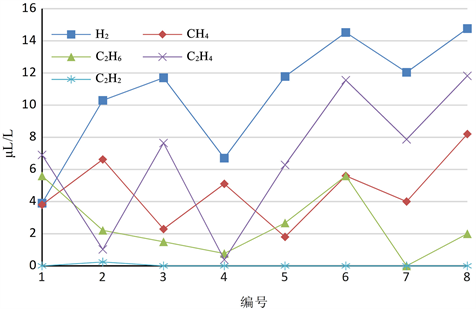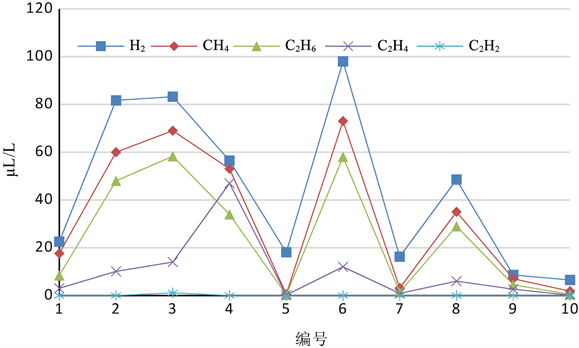灰色关联分析在变压器DGA缺码数据中的应用研究Application Research of Grey Relational Analysis in Transformer DGA Code Absence Data

• 全文下载: PDF(728KB)    PP.325-331   DOI: 10.12677/JEE.2018.64037
• 下载量: 247  浏览量: 683

Three-ratio method is one of the effective methods for latent fault diagnosis of power transfor-mers, but there is a lack of code in this method. According to the DGA data collected in this paper, it is found that the variation trend of the characteristic gas in the same fault samples is the same, and the variation trend of different fault features is obviously different. In this paper, by excavate the changing trend of each data in DGA, using the grey relational theory and using the DGA fault identification method based on grey relational analysis, the fault diagnosis of code absence power transformers in DGA is carried out. It makes up for the deficiency of the three ratio method and improves the accuracy of transformer fault diagnosis. The research results have important theoretical value and practical value of engineering. It can be popularized vigorously and bring great economic benefits to the power system and the society.

1. 引言

2. 故障气体规律Table 1. Data and fault type of the code 012 (uL/L)Figure 1. Data of the code 012

3. 灰色关联度方法简介

${X}_{i}=\left({x}_{i}\left(1\right),{x}_{i}\left(2\right),\cdots ,{x}_{i}\left(n\right)\right)$$i=0,1,2,\cdots ,m$

$\gamma \left({x}_{0}\left(k\right),{x}_{i}\left(k\right)\right)=\frac{\underset{i}{\mathrm{min}}\underset{k}{\mathrm{min}}{\Delta }_{0i}\left(k\right)+\xi \underset{i}{\mathrm{max}}\underset{k}{\mathrm{max}}{\Delta }_{0i}\left(k\right)}{{\Delta }_{0i}\left(k\right)+\xi \underset{i}{\mathrm{max}}\underset{k}{\mathrm{max}}{\Delta }_{0i}\left(k\right)}$ (1)

${x}_{i}\left(k\right)$${x}_{0}\left(k\right)$ 的关联度系数， ${\Delta }_{0i}\left(k\right)$${x}_{i}\left(k\right)$${x}_{0}\left(k\right)$ 两点之间的绝对差， ${\Delta }_{0i}\left(k\right)=|{x}_{0}\left(k\right)-{x}_{i}\left(k\right)|$ $\xi \in \left[0,1\right]$ 为分辨系数。

$\gamma \left({x}_{0},{x}_{i}\right)=\frac{1}{n}\underset{k=1}{\overset{n}{\sum }}\gamma \left({x}_{0}\left(k\right),{x}_{i}\left(k\right)\right),k=0,1,2,\cdots ,n$ (2)

4. 故障诊断方法应用步骤Table 2. The proportion of fault gasesTable 3. Data and fault type of the code 011 (uL/L)

$\gamma {\left(i\right)}_{\psi }=\frac{1}{n-1}\underset{j=1,j\ne i}{\overset{n-1}{\sum }}\gamma {\left(i,j\right)}_{\psi }$

$\gamma {\left(\zeta \right)}_{\psi }=\frac{1}{n}\underset{i=1}{\overset{n}{\sum }}\gamma {\left(i\right)}_{\psi }$

$\gamma \left(\zeta \right)=1-\frac{1}{5}\underset{\psi }{\sum }|\gamma {\left(\zeta \right)}_{\psi }-\gamma {\left(0\right)}_{\psi }|$

5. 实例验证分析Figure 2. Trend of low temperature overheating fault about code 011 in partial discharge dataTable 4. Fault diagnosis results of the code 000 used by grey relational analysis

6. 结论

  杜正聪, 牛高远. 基于加权模糊聚类算法的变压器故障诊断方法[J]. 高压电器, 2014, 50(4): 42-48.  杨延方, 张航, 黄立滨, 等. 基于改进主成分分析的电力变压器潜伏性故障诊断[J]. 电力自动化设备, 2015, 35(6): 149-165.  GB/T7252-2001 变压器油中溶解气体分析和判断导则[S].  宋斌, 刘志雄, 李恩文, 等. 基于负关联度的DGA故障诊断分析[J]. 电网技术, 2015, 39(9): 2627-2632.  葛乐, 陆文伟, 周志成, 等. 基于改进熵权法和灰色关联分析的变压器故障诊断[J]. 电测与仪表, 2016, 53(12): 46-51.  蔡金锭, 黄云程. 基于灰色关联诊断模型的电力变压器绝缘老化研究[J]. 高电压技术, 2015, 41(10): 3296-3301.  Singh, J. and Sood, Y.R. (2007) Dissolved Gas Analysis for Power Transformers. Electr. India.  宋臻杰. 基于灰色关联分析的变压器油纸绝缘状态评估研究[D]: [硕士学位论文]. 成都: 西南交通大学, 2017.  Bigdeli, M., Vakilian, M. and Rahimpour, E. (2012) Transformer Winding Faults Classification Based on Transfer Function Analysis by Support Vector Machine. IET Electric Power Applications, 6/5, 268-276. https://doi.org/10.1049/iet-epa.2011.0232  张安平. 基于熵权优化灰色关联度方法的电力变压器故障诊断研究[D]: [硕士学位论文]. 南京: 南京理工大学. 2016.  Dong, L., Xiao, D., Liang, Y., et al. (2008) Rough Set and Fuzzy Wavelet Neural Network Integrated with Least Square Weighted Fusion Algorithm Based Fault Diagnosis Research for Power Transformers. Electric Power Systems Research, 78, 129-136. https://doi.org/10.1016/j.epsr.2006.12.013  刘思峰. 灰色系统理论及其应用[M]. 北京: 科学出版社, 2008: 22-65.  孙鹏霄. 灰色关联方法的分析与应用[J]. 数学的实践与认识, 2014, 44(1): 97-101.  张卫华, 苑津莎, 张铁峰, 等. 应用B样条理论改进的变压器三比值故障诊断方法. 中国电机工程学报, 2014, 34(24): 4129-4135.  Zhao, J.Y., Zheng, R.R., Li, J.P. (2009) Transformer Fault Diagnosis Based on Homotopy BP Algorithm. 9th International Conference on Electronic Measurement & Instruments (ICEMI), 4, 622-626. https://doi.org/10.1109/ICEMI.2009.5274664  李硕, 赵峰. 基于熵权优化加权灰色关联度的变压器故障诊断方法[J]. 变压器, 2013, 37(1): 136-142.  Barbosa, F.R. and Braga, A.P.S. (2012) Application of an Artificial Neural Network in the Use of Physicochemical Properties as a Low Cost Proxy of Power Transformers DGA Data. IEEE Transactions on Dielectrics and Electrical Insulation, 19, 239-246. https://doi.org/10.1109/TDEI.2012.6148524  张东波, 徐瑜 王耀南. 主动差异学习神经网络集成方法在变压器DGA故障诊断中的应用[J]. 中国电机工程学报, 2010, 30(22): 64-70.  邹杰慧. 基于新型编码隶属函数的变压器故障模糊诊断法[J]. 电力系统自动化设备, 2010, 30(7): 88-91.